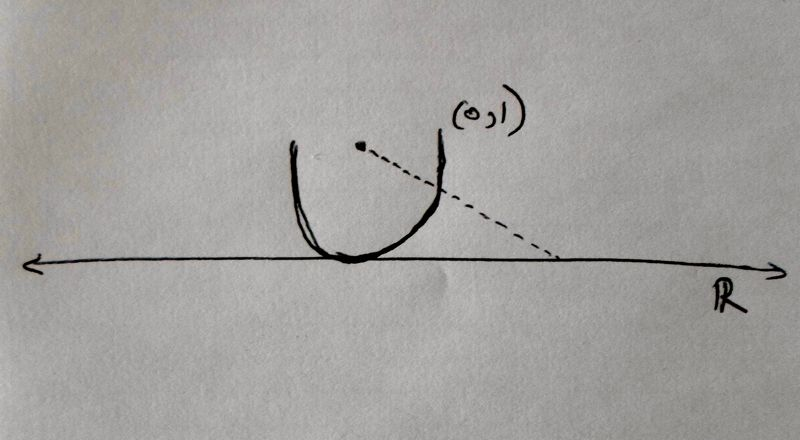# Story

The seeds of Cantor’s ideas can be found in others’ work.

The one-to-one correspondence between $$(0,1)$$ and $$\mathbb{R}$$ that we describe in our explanations does not have any interesting backstory. There are many possible functions one could chose between $$(0,1)$$ and $$\mathbb{R}$$ that would yield a one-to-one correspondence. The one we use in the Short and In-Depth explanations was chosen because it is easy to write down and explain.

A different one, more pictorial and less algebraic, would be "defined" by the following picture. It shows how every point on a half-circular version of $$(0,1)$$ can be put in correspondence with every point on the real line $$\mathbb{R}$$, by drawing a line out from the center point.(Imagine putting a translucent globe on a piece of paper, putting a lightbulb at the center of the globe, and tracing the map out onto the paper. The picture above is a lower-dimensional version of that assignment rule.)

The mythology of mathematicians includes many constructed geniuses, and Cantor is one. But history is never as straightforward as legend. Just as Hendrik Lorentz and other physicists were using the term "relativity" to refer to the same thing as Einstein, years before Einstein popularized it, there are threads of Cantor's ideas buried in his past.

The notion of one-to-one correspondence is the insight on which all of Cantor's infinite set theory rests. He never claimed to have been the first to reach this insight, and indeed he wasn't. The first may have been Galileo Galilei, in 1638, who said the following in "Dialogues Concerning the Two New Sciences":

"If I should ask further how many squares there are, one might reply truly that there are as many as the corresponding number of roots, since every square has its own root and every root has its own square, while no square has more than one root and no root more than one square."

Another unattributed predecessor of Cantor was Bernhard Bolzano (1781-1848). After losing his job as the chair of the department of philosophy of religion at the University of Prague, in 1824 (for, among other things, teaching that war would be deemed morally unacceptable by future generations), Bolzano quietly worked as a mathematician. His posthumous publication "Paradoxes of Infinity", argues that any two intervals of real numbers -- for example $$(0,1)$$ and $$(0,5)$$ -- have the same number of elements. He defines a function -- in this case $$f(x) = 5x$$ -- and shows that it is a one-to-one correspondence.

By clarifying the notion of one-to-one correspondence, and simultaneously identifying the inevitable paradoxes that begin to emerge from it, Bolzano foreshadowed the revolutionary work of Cantor.

Top